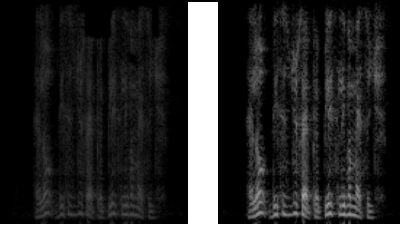# Spectrogram to RGB pictures for Resnet: faint image with mp3 files

Hello,
I am trying to generate pictures from audio spectrogram. I couldn’t find specific examples on internet and I attempted to put together a solution myself.

I managed to implement an algorithm that can generate pictures passing files encoded mp3 or wav.
At high level everything seems to work ok for Wav files but for mp3 I seem to generate a picture where the spectrum is faint (compared to the one generated by the wav file).
I generated the files using Audacity and I saved the track to mp3 or wav.
Below is the code. I am using Jupiterlab on Sagemaker for the runtime environment.

This seems to be a standard use case in audio classification modelling. Can somebody help explain the reason behind this and whether there is any resource that could have code that can convert audio to RGB pictures for Resnet ingestion ?

``````import torch
import torchaudio
import matplotlib.pyplot as plt

def normalize_input(tensor):
# Subtract the mean, and scale to the interval [-1,1]
tensor_minusmean = tensor - tensor.mean()
return tensor_minusmean/tensor_minusmean.abs().max()

filename = "/home/ec2-user/SageMaker/GiuseppeProjects/GiuseppeTest.mp3"

#waveform = np.delete(waveform, (1), axis=0)
waveform = normalize_input(waveform)
print("Shape of waveform: {}".format(waveform.size()))
print("Sample rate of waveform: {}".format(sample_rate))

#print(waveform)

plt.figure()
plt.plot(waveform.t().numpy())
plt.show()
``````
``````import sys
from torchvision import transforms
import torchvision
from skimage.util import img_as_ubyte
from skimage import exposure
from sklearn import preprocessing
from PIL import Image
import numpy as np

transform_spectra = transforms.Compose([
transforms.ToPILImage(),
transforms.Resize((224,224)),
transforms.RandomVerticalFlip(1)
])

def make0min(tensornd):
tensor = tensornd.numpy()
res = np.where(tensor == 0, 1E-19 , tensor)

def normalize(tensor):
tensor_minusmean = tensor - tensor.mean()
return tensor_minusmean/tensor_minusmean.abs().max()

def normalize_nd(tensor):
tensor_minusmean = tensor - tensor.mean()
return tensor_minusmean/np.absolute(tensor_minusmean).max()

def spectrogrameToImage(waveform):
specgram = torchaudio.transforms.Spectrogram(n_fft=400, win_length=None, hop_length=None, pad=0,window_fn=torch.hann_window, power=2, normalized=True, wkwargs=None)(waveform )
specgram= make0min(specgram)
specgram = specgram.log2()[0,:,:].numpy()

np.set_printoptions(linewidth=300)
np.set_printoptions(threshold=sys.maxsize)

specgram= normalize_nd(specgram)
specgram = img_as_ubyte(specgram)
specgramImage = transform_spectra(specgram)
return specgramImage

def print_spec(spec):
torch.set_printoptions(linewidth=150)
torch.set_printoptions(profile="full")
#spec = torchvision.transforms.ToTensor()(spec)
spec = np.array(spec)
#print (spec)

waveform = normalize(waveform)
spec = spectrogrameToImage(waveform)
spec = spec.convert('RGB')

plt.figure()
plt.imshow(spec)
``````I seem to have achieved better results by eliminating the first 10 data points of the spectrogram. It looks like there is a clear difference in the spectrogram for the first data points which causes this problem when normalizing the picture.
I assume this is something with the difference between MP3 and WAV that anything to do with Spectrogram ?

Have you printed a sample from each waveform? Printing some statistics like mean, std dev, range, of each, and some information about the difference would also help. I expect the difference to come from the waveform and not the spectrogram.